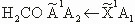# Lecture Notes

(S) = Supplemental notes.

LEC # TOPICS LECTURE NOTES
0 General information (PDF)
1 Matrices are useful in spectroscopic theory (PDF)
1 (S) Spectroscopic notation, good quantum numbers, perturbation theory and secular equations, non-orthonormal basis sets, transformation of matrix elements of any operator into perturbed basis set (PDF)
2 Coupled harmonic oscillators: truncation of an infinite matrix (PDF)
2 (S) Matrix solution of harmonic oscillator problem, derivation of heisenberg equation of motion, matrix elements of any function of X and P (PDF)
3 Building an effective hamiltonian (PDF)
3 (S) Anharmonic oscillator, vibration-rotation interaction, energy levels of a vibrating rotor (PDF)
4 Atoms: 1e- and alkali (PDF)
5 Alkali and many e- atomic spectra (PDF)
6 Many e- atoms (PDF)
7 How to assign an atomic spectrum (PDF)
8 The Born-Oppenheimer approximation (PDF)
8 (S) Excerpts from the spectra and dynamics of diatomic molecules (PDF) ( Field, R. W., and H. Lefebvre-Brion. The Spectra and Dynamics of Diatomic Molecules. Burlington, MA: Elsevier Academic Press, 2004. ISBN: 9780124414556. Courtesy of Elsevier. Used with permission.)
9 The Born-Oppenheimer approach to transitions (PDF)
10 The Born-Oppenheimer approach to transitions II (PDF)
11 Pictures of spectra and notation (PDF)
12 Rotational assignment of diatomic electronic spectra I (PDF)
13 Laser schemes for rotational assignment first lines for Ω’, Ω" assignments (PDF)
14

Definition of angular momenta and | A α MA >

Evaluation of ĤROT

(PDF)
14 (S) Rotation and angular momenta (PDF)
15 2∏ and 2∑ matrices (PDF)
16 Parity and e/f basis for 2∏, 2± (PDF)
17 Hund’s cases: 2∏, 2± examples (PDF)
17 (S) Energy level structure of 2∏ and 2∑ states, matrix elements for 2∏ and 2∑ including ∏ ~ ∑ perturbation, parity (PDF)
18 Perturbations (PDF)
18 (S) A model for the perturbations and fine structure of the ∏ states of CO, factorization of perturbation parameters, the electronic perturbation parameters (PDF)
19 Second-order effects (PDF)
19 (S) Second-order effects: centrifugal distortion and Λ-doubling (PDF)
20 Transformations between basis sets: 3-j, 6-j, and Wigner-Eckart theorem (PDF)
21 Construction of potential curves by the Rydberg-Klein-Rees method (RKR) (PDF)
22 Rotation of polyatomic molecules I (PDF)
22 (S) Energy levels of a rigid rotor, energy levels of an asymmetric rotor (PDF)
23 Asymmetric top (PDF)
23 (S) Energy levels of a rigid rotor, energy levels of an asymmetric rotor (PDF)
24 Pure rotation spectra of polyatomic molecules (PDF)
24 (S) Energy levels of a rigid rotor (PDF)
25 Polyatomic vibrations: normal mode calculations (PDF)
26 Polyatomic vibrations II: s-vectors, G-matrix, and Eckart condition (PDF)
27 Polyatomic vibrations III: s-vectors and H2O (PDF)
28 Polyatomic vibrations IV: symmetry (PDF)
29 A sprint through group theory (PDF)
30 What is in a character table and how do we use it? (PDF)
31 Electronic spectra of polyatomic molecules (PDF)
32

Thetransition

(PDF)
33 Vibronic coupling (PDF)
33 (S) Time-independent Schrodinger equation for a molecular system (PDF)
34 Wavepacket dynamics (PDF)
35 Wavepacket dynamics II
36 Wavepacket dynamics III (PDF)

#### Learning Resource Types

assignment_turned_in Problem Sets with Solutions